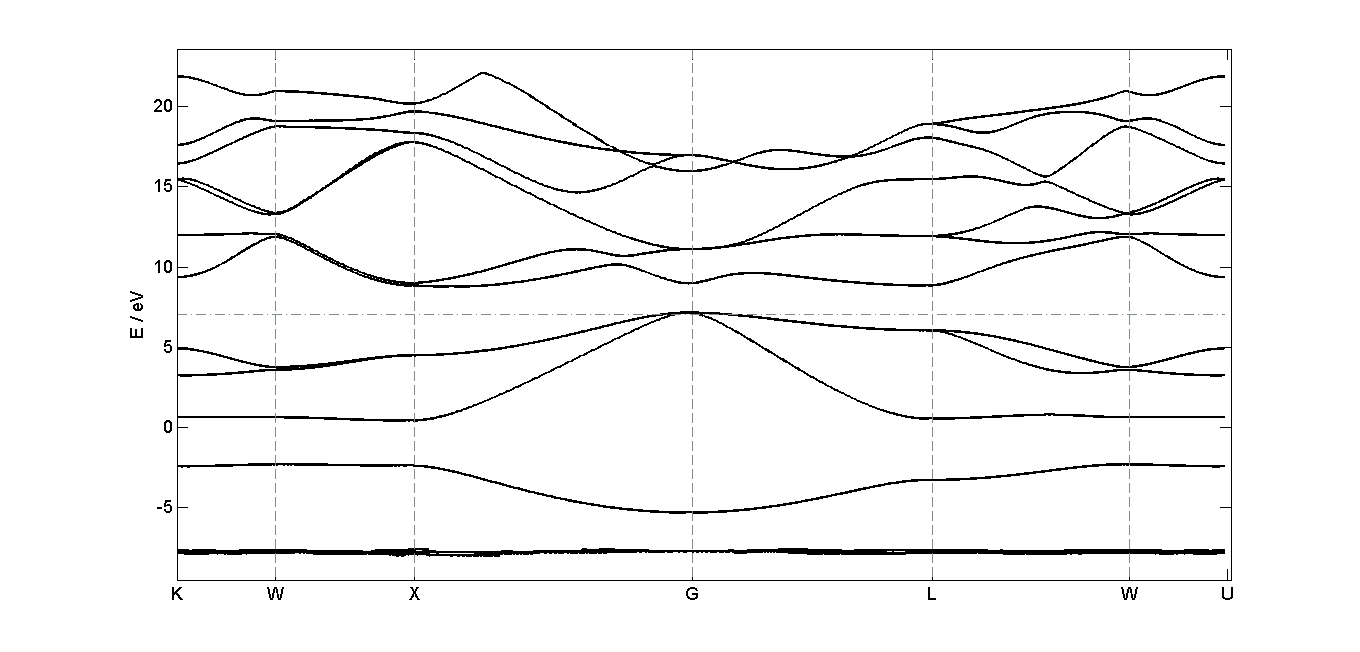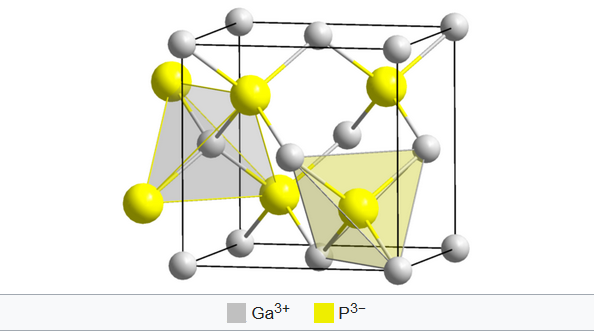## Bandstructure of gallium phosphide (GaP)

The electron bandstructure for gallium phosphide (zincblende structure) was calculated using the program Quantum Espresso (version 5.3.0). GaP is a compound semiconductor material with an indirect bandgap of 2.26 eV. The calculated bandgap (1.82 eV) is a little smaller than comparative values. (cf. ioffe) .Annotation:
The outcoming data of Quantum Espresso were prepared by a Matlab-Script. The script allows to choose a order of symmetry points and plots the corresponding bandstructure. Also the density of states can be plotted.

Matlab-Script:
Input files:

Output files:
GaP_data.zipZincblende structure of GaP Source: Wikipedia

Density of states